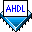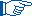## Arithmetic Expressions

 See AlsoArithmetic expressions can be used to define evaluated functions in Define Statements, constants in Constant Statements, and parameter values in Parameters Statements. They can also be used as the delimiters of group ranges. Example

You can use the following arithmetic operators and comparators in an arithmetic expression to perform basic operations on the numbers and constants in the expression:

Operator/ Comparator: Example: Description: Priority:
`+` (unary) `+1` positive 1
`-` (unary) `-1` negative 1
`!` `!a` NOT 1
`^` `a ^ 2` exponent 1
`MOD` `4 MOD 2` modulus 2
`DIV` `4 DIV 2` division 2
`*` `a * 2` multiplication 2
`LOG2` `LOG2(4-3)` logarithm base2 2
`+` `1+1` addition 3
`-` `1-1` subtraction 3
`==` (numeric) `5 == 5` numeric equality 4
`==` (string) `"a" == "b"` string equality 4
`!=` `5 != 4` not equal to 4
`>` `5 > 4` greater than 4
`>=` `5 >= 5` greater than or equal to 4
`<` `a < b+2` less than 4
`<=` `a <= b+2` less than or equal to 4
`&`
`AND`
`a & b`
`a AND b`
AND 5
`!&`
`NAND`
`1 !& 0`
`1 NAND 0`
NAND (AND inverter) 5
`\$`
`XOR`
`1 \$ 1`
`1 XOR 1`
XOR (exclusive OR) 6
`!\$`
`XNOR`
`1 !\$ 1 `
`1 XNOR 1`
XNOR (exclusive NOR) 6
`#`
`OR`
`a # b`
`a OR b`
OR 7
`!#`
`NOR`
`a !# b`
`a NOR b`
NOR (OR inverter) 7
`? :` `(5<4) ? "five":"four"` ternary 8

The unary plus (`+`) and minus (`-`) are prefix operators. The `+` operator does not affect its operand, but you can use it for documentation purposes (that is, to indicate a positive number).You can also use the predefined evaluated functions `USED`, `CEIL`, and `FLOOR` in arithmetic expressions.

The following rules apply to all arithmetic expressions:

• An arithmetic expression must resolve to a string or non-negative number.

• When the result of `LOG2` is not an integer, the result is automatically rounded up to the next integer. ExampleThe arithmetic operators that are supported in arithmetic expressions are a superset of the arithmetic operators supported in Boolean expressions, which are described in Arithmetic Operators in Boolean Expressions.